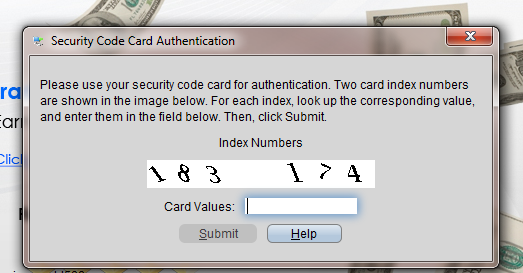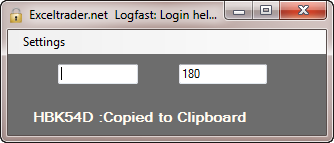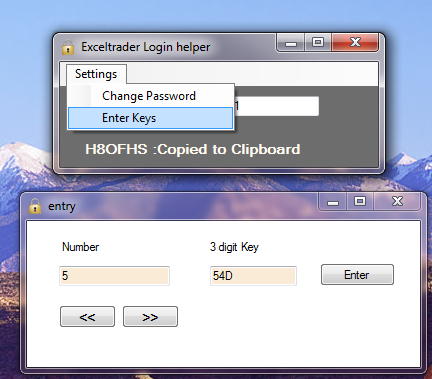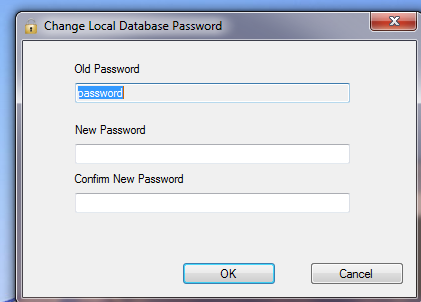## Earnings_2016.xls

### Description:

earnings_2016.xls is a newer/better version of the older earnings.xls Excel file that retrieves earnings dates from various sources (requires Windows).

### Usage:

2. Enter Stock Symbols in Column A
3. Press “Get Earnings”Notes:
One of the most downloaded files on exceltrader.net is the original version of earnings.xls. The earnings dates are retrieved from various sites that often change. After a source made a change, it was common for earnings.xls to stop working until I had time to update it.

earnings_2016.xls (File Size:54.0 KiB, Total downloads: 4824, Last Updated: January 25, 2016) is a complete do over with an attempt to make it very easy for a VBA beginner to make a change (or customize) and get things working again without having to wait for me to do it. In addition, it will be faster for me to update as needed.

As an example, on any page that contains an Earnings Date, view the html source and find a unique string near the date. On line 6 below, a value that is currently working on yahoo is “Earnings Date:”. The next string we’ll use is yfnc_tabledata1″> between the first string and the date and that’s all we need to find the date.If the site changes the “Earnings Date:” to something like “Earn Date:”, then the code will stop working. Most likely it can be fixed simply by changing line six below in earningsmodule.bas. (Most likely the classes won’t need to be modified.)

The exported module and classes are available on github. If you spend time making additions or fixes that you would like to share, consider a pull request.

## A small program to assist IB login for users with Security cards.

IB now provides account holders with a plastic security card that has 224 numbers (1-224)  listed next to each number’s corresponding three digit unique key. To login, the user must look up two numbers provided by IB at login and then find and type in the corresponding two keys. Many find the process cumbersome and opt out of being required to use the security device. Opting out requires the account holder to sign forms giving up certain rights and is not a great choice considering that IB for some reason limits password maximum length to 8 characters.LogFast_et is a small program to assist the cumbersome process of logging in. Once the program is set up, The user opens the small program, enters the 2 numbers displayed by IB and the resulting code is copied automatically to the clipboard so that it can be pasted in  IB’s login area to complete the login.

Initial Setup: Download and install the program. To setup the program, there is a form in place available from the settings menu to enter the values from the security card. The setup should take most people around 20 minutes to enter all 224 entries. Fortunately you’ll only have to do it once.Below is a short video showing the program in action.  Please use the comment form below for any questions, comments, feature requests.

## MACD VBA

This post includes links to two example xls files that show both VBA and Formula based calculations for the indicator MACD or Moving Average Convergence Divergence.

Both files contain the exact same set of Open High Low Close data. The formula based version is easier to understand and serves as a way to verify that the VBA code that I wrote is correct. Both methods give the same result.  The major benefit of using VBA is that the parameters for MACD can be easy changed from the input boxes. In addition the VBA method shows only the final result rather than take up five columns.

The formula version can be downloaded here. Below is the code from the VBA based version.

Dim EMAslow As Double, EMAfAst As Double, ws As Worksheet, LR As Integer
Dim eMaF() As Double, eMaS() As Double, EMAdif(), emaPer() As Double, MacDper As Double, coUnt As Integer
Dim DataRange As Range
Dim ExPSlowWeight As Double
Dim ExPFastWeight As Double
Dim PerWeight As Double
'''''''''''''''''''''''''''''''''''''''''''''''''''''''''''''''''''''''''''''''''''''''''''
''  The below three lines are the MACD settings.                                         ''
''  The Values can either be changed here or uncomment the inputbox lines to be prompted.''
'''''''''''''''''''''''''''''''''''''''''''''''''''''''''''''''''''''''''''''''''''''''''''
EMAslow = 13 'InputBox(Prompt:="Enter Macd Slow settings.", Title:="MACD SLOW", Default:="13")
EMAfAst = 5 'InputBox(Prompt:="Enter Macd Fast settings.", Title:="MACD Fast", Default:="5")
MacDper = 6 'InputBox(Prompt:="Enter Macd Period settings.", Title:="MACD Period", Default:="6")
ExPSlowWeight = 2 / (EMAslow + 1)
PerWeight = 2 / (MacDper + 1)
ExPFastWeight = 2 / (EMAfAst + 1)
Set ws = ThisWorkbook.Worksheets("VBA") 'or use exact sheet name for example ThisWorkbook.worksheet("Sheet1")
'slow
With ws
LR = .Cells(Rows.coUnt, "A").End(xlUp).Row
For Each DataRange In ws.Range(.Cells(2, "A"), .Cells(LR, "A"))
coUnt = DataRange.Row + 1
'fill the eMA slow Array
ReDim Preserve eMaS(1 To coUnt)
If coUnt = EMAslow + 1 Then
'get the first value which is the Simple Moving average
eMaS(coUnt) = Application.Average(ws.Range(.Cells(2, "E"), .Cells(coUnt, "E")))
ElseIf coUnt > EMAslow Then
eMaS(coUnt) = (.Cells(coUnt, "E") * ExPSlowWeight) + (eMaS(coUnt - 1) * (1 - ExPSlowWeight))
End If
Next DataRange
'fast
For Each DataRange In ws.Range(.Cells(2, "A"), .Cells(LR, "A"))
coUnt = DataRange.Row + 1
'fill the eMA slow Array
ReDim Preserve eMaF(1 To coUnt)
If coUnt = EMAfAst + 1 Then
'get the first value which is the Simple Moving average
eMaF(coUnt) = Application.Average(ws.Range(.Cells(2, "E"), .Cells(coUnt, "E")))
ElseIf coUnt > EMAfAst Then
eMaF(coUnt) = (.Cells(coUnt, "E") * ExPFastWeight) + (eMaF(coUnt - 1) * (1 - ExPFastWeight))
End If
Next DataRange
For coUnt = EMAslow + 1 To UBound(eMaF)
Next coUnt
'MacD Period
Dim x As Integer, y As Integer, z As Integer, Avee As Double
y = EMAslow + MacDper - 1
For x = y To UBound(EMAdif) - 2
'get the SMA for first value
If x = y Then
For z = EMAslow + 1 To EMAslow + MacDper  '(EMAslow + MacDper - 1)
Next z
ReDim emaPer(x To LR)
emaPer(x) = Ave / MacDper
ElseIf x > y Then
emaPer(x) = (EMAdif(x + 1) * PerWeight) + (emaPer(x - 1) * (1 - PerWeight))
End If
Next x
x = Empty: y = Empty: z = Empty
x = LBound(emaPer)
y = UBound(emaPer)
For z = x To y - 1
.Cells(z + 1, "J") = EMAdif(z + 1) - emaPer(z)
Next z
End With

## Data Converter to Convert OHLC Data to a larger timeframe.

If you have ever wanted to take your existing Open High Low Close historical trading data and convert it into larger time frame, the following Excel VBA based data converter I wrote may do the trick.DataConverter.xls allows you to configure the Number of OHLC bars that should be condensed down into a single bar. You also can list the file name, sheet, and columns where your data is located.  The data will almost instantly be transformed into the higher time frame you have requested.

Instructions: